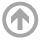Naval Architecture & Ocean Engineering# EN222: Engineering Mechanics with Marine Applications II

## Catalog Description

### EN222: Engineering Mechanics with Marine Applications II (3-2-4)

The second course in a two semester sequence covering the principles of engineering mechanics of rigid and deformable bodies for naval architecture students. Topics in the second course include: generalized Hooke's Law and states of stress, structural failure theories, motion of a point, energy methods, impact loadings, momentum methods, planar kinematics of rigid bodies, energy and momentum in rigid body dynamics, and single DOF vibration analysis.

### Textbook

• Mechanics of Materials (Vable, 2016)
• Engineering Mechanics: Dynamics (Hibbeler, 2016)

### Goals

1. Calculate the stresses and deflections in a beam under given loading conditions.
2. Solve for the loading capacity of columns, given geometry and end conditions.
3. Construct free-body and kinetic diagrams for particle or rigid-body motions and apply them to solve for the equations of motion.
4. Solve problems involving particles, rigid bodies, and dynamic impact using work-energy and impulse-momentum principles.
5. Determine the equation of motion for a single DOF oscillator and solve for motion amplitude, velocity, acceleration, and phase.
6. Create a computer program to solve typical engineering mechanics problems.

### Prerequisites

• EN221, Engineering Mechanics with Marine Applications I

### Class Topics

1. Beam Bending
2. Column Buckling
3. Straight-Line, Curvilinear, and Angular Motion
4. Work and Energy
5. Impulse and Momentum
6. Energy Methods
7. Rigid-Body Motion
8. Harmonic Motion and Oscillation

### Laboratory Projects

1. Problem Solving Using Excel – Beam Bending
2. Problem Solving Using Excel – Column Buckling
3. Problem Solving Using Excel – Equations of Motion (SOLVER)
4. Impacts of Structures
5. Dynamic Ballasting
6. Roll Motion in Waves